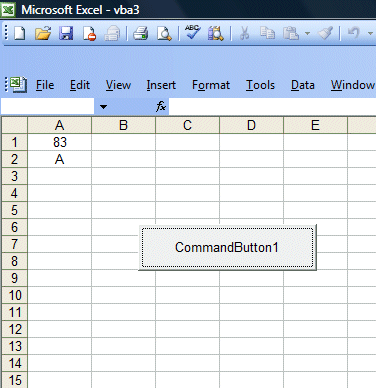# Excel VBA Lesson 12: Using If…..Then….Else

#### <<Lesson 11>> [Contents] <<Lesson 13>>

In this lesson, you will learn how to create Excel VBA code that can make a decision. Decision-making process is an important part of Excel VBA  programming because it can help to solve practical problems intelligently so that it can provide useful feedback to the users.In Excel VBA, decision making involves the use of the  If..Then…Else syntax to process data and display the output based on certain conditions. To effectively control the VB program flow, we shall use If…Then…Else statement together with the conditional operators and logical operators. These operators are shown in Table 12.1 and Table 12.2 respectively.Table 12.1:Conditional OperatorsTable 12.2 Logical Operators

We shall demonstrate the usage of If….Then…Else with the following example.In this program, we place the command button1 on the MS Excel spreadsheet and go into the VB editor by clicking on the button. At the VB editor, enter the program code as shown below. We use the RND function to generate random numbers. In order to generate random integers between 0 and 100, we combined the syntax Int(Rnd*100). For example, when Rnd=0.6543, then Rnd*100=65.43, and Int(65.43)=65. Using the statement cells(1,1).Value=mark will place the value of 65 into cell(1,1).

Now, based on the mark in cells(1,1), I use the If…….Then….Elseif statements to put the corresponding grade in cells(2,1). So, when you click on command button 1, it will put a random number between 1 and 100 in cells(1,1) and the corresponding grade in cells(2,1).

The Code

Private Sub CommandButton1_Click()
Dim mark As Integer
Dim grade As String
Randomize Timer
mark = Int(Rnd * 100)
Cells(1, 1).Value = mark
If mark < 20 And mark >= 0 Then
Cells(2, 1).Value = grade
ElseIf mark < 30 And mark >= 20 Then
Cells(2, 1).Value = grade
ElseIf mark < 40 And mark >= 30 Then
Cells(2, 1).Value = grade
ElseIf mark < 50 And mark >= 40 Then
Cells(2, 1).Value = grade
ElseIf mark < 60 And mark >= 50 Then
Cells(2, 1).Value = grade
ElseIf mark < 70 And mark >= 60 Then
Cells(2, 1).Value = grade
ElseIf mark < 80 And mark >= 70 Then
Cells(2, 1).Value = grade
ElseIf mark <= 100 And mark >=80 Then